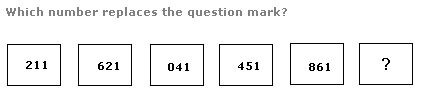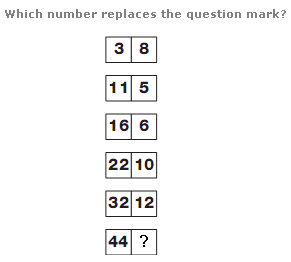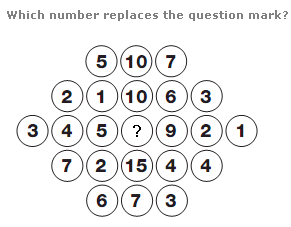# Puzzles - Number puzzles

### Exercise :: Number puzzlesAnswer : 281 Explanation : Working from top to bottom, numbers follow the sequence of multiples of 14, from 112 to 182, with each value being written back to front.Answer : 20 Explanation : Working from top to bottom, the sum of the numbers in each row is put in the left hand box on the row below, and the difference between the two numbers in each row is put in the right hand box on the row below.Answer : 22 Explanation : On each row of the diagram, the value in the centre of each row equals the sum of the other numbers in the row, subtracting 2.